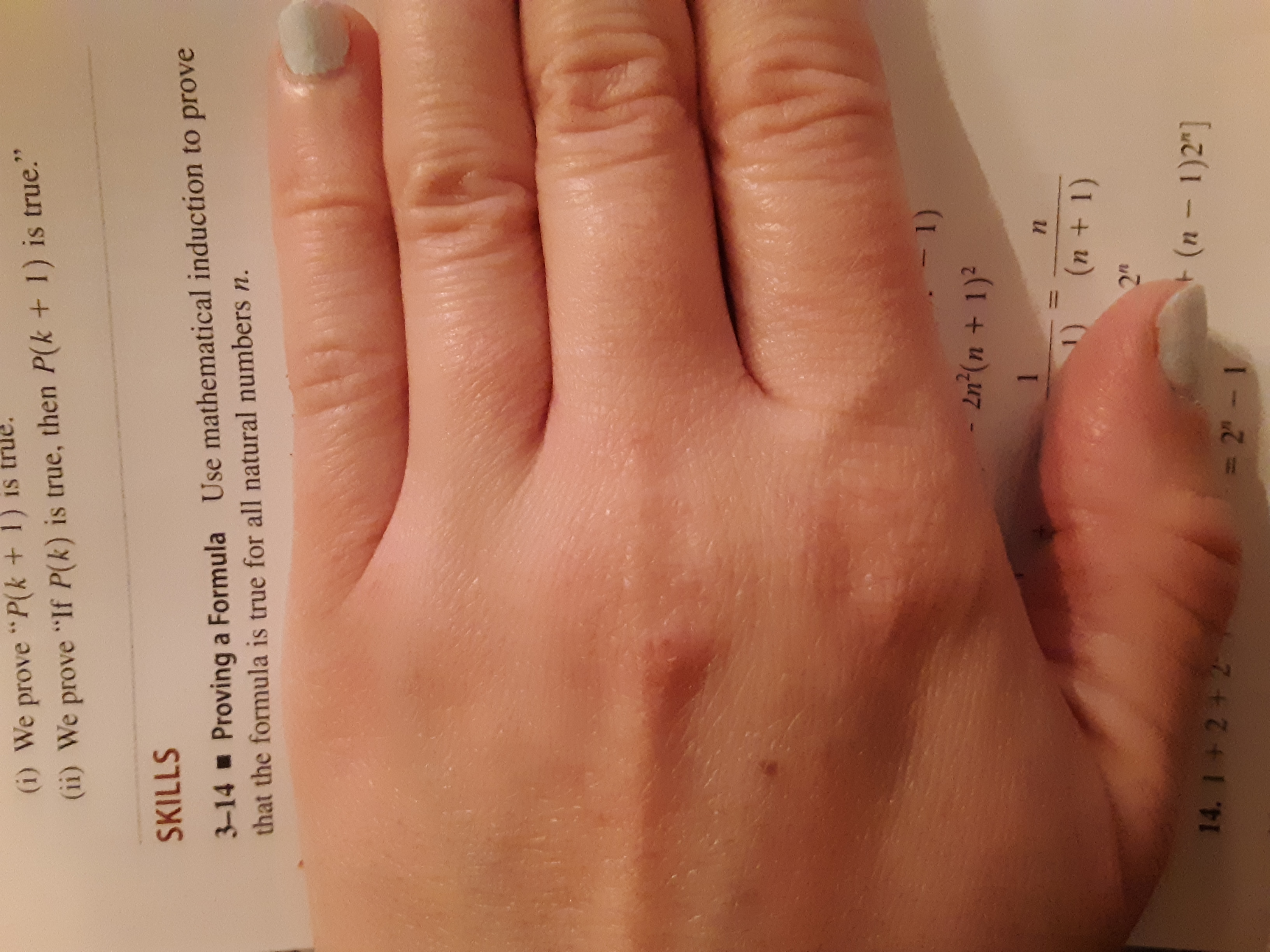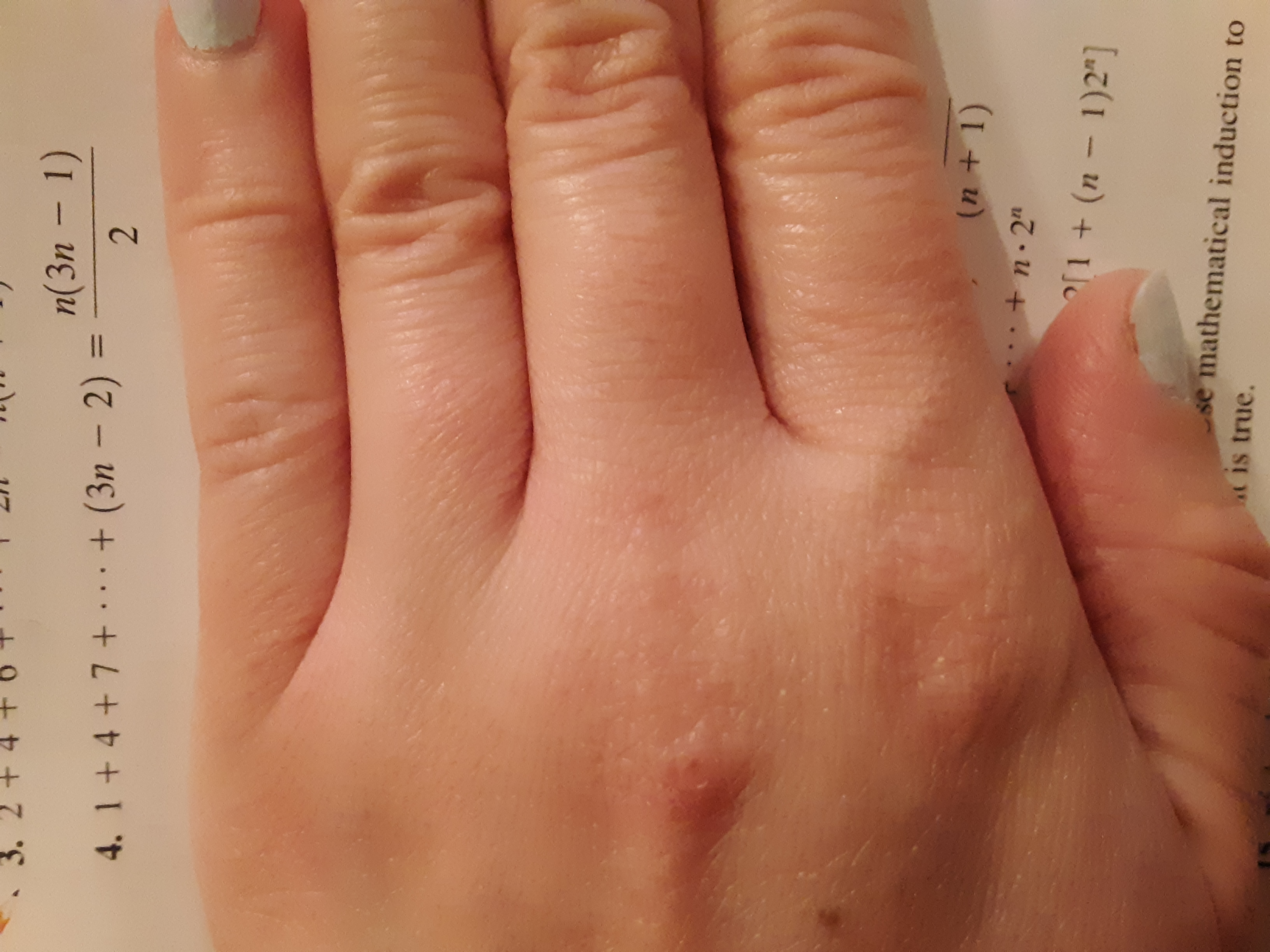# (i) We prove "P(k + 1)(ii) We prove "If P(k) is true, then P(k+ 1) is true."SKILLS3-14 Proving a Formula Use mathematical induction to provethat the formula is true for all natural numbers n.- 1)2n (n + 1)21n(n+1)2"(n - 1)2"14. I + 2 +2-2 1 n(3n - 1)+ (3n- 2)24. 1+ 4+7+(n + 1)+n.2"[1 (n - 1)2"]se mathematical induction tot is true.++

Question
2 views

See images below....help_outlineImage Transcriptionclose(i) We prove "P(k + 1) (ii) We prove "If P(k) is true, then P(k+ 1) is true." SKILLS 3-14 Proving a Formula Use mathematical induction to prove that the formula is true for all natural numbers n. - 1) 2n (n + 1)2 1 n (n+1) 2" (n - 1)2" 14. I + 2 +2 -2 1 fullscreenhelp_outlineImage Transcriptionclosen(3n - 1) + (3n- 2) 2 4. 1+ 4+7+ (n + 1) +n.2" [1 (n - 1)2"] se mathematical induction to t is true. + + fullscreen
check_circle

Step 1

The given formula to be proved by the method of mathematical induction for all natural numbers n is,

Step 2

Prove that the statement is true when the value of n is 1.

Step 3

Assume that the formula is true when the ...

### Want to see the full answer?

See Solution

#### Want to see this answer and more?

Solutions are written by subject experts who are available 24/7. Questions are typically answered within 1 hour.*

See Solution
*Response times may vary by subject and question.
Tagged in

### Other